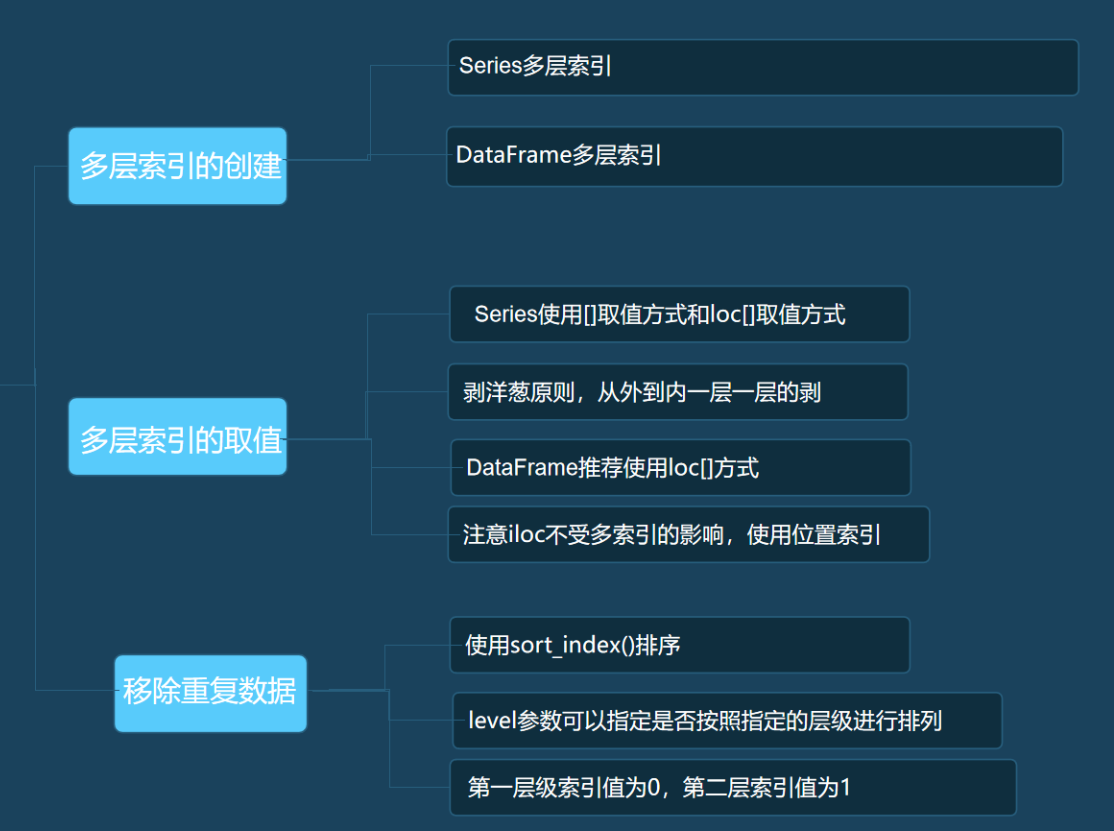# python数据分析004_多层索引的取值和排序

1. np.random.randint(0,100,size=(6,3))是使用numpy中的随机模块random中，生成随机整数方法randint，里面的参数size是指定生成6行3列的数据，并且每个数字的范围在0到100之间。

• import pandas as pd
• import numpy as np
• data = np.random.randint(0,100,size=(6,3))
• names = ['张三','李四','王五']
• exam = ['期中','期末']
• index = pd.MultiIndex.from_product([names,exam])
• df = pd.DataFrame(data,index=index,columns=['Java','Web','Python'])
• print(df)

2.pd.MultiIndex.from_product()构建索引的方式, 确定每一层索引的值什么，然后以列表的形势传给from_product()方法即可。

3.[names,exam]列表中的位置不同，产生的索引也会不同。from_product([exam,names])会将列表中第一个元素作为最外层索引，依次类推

1.loc使用的是标签索引，iloc使用的是位置索引。但是，iloc的取值并不会受多层索引影响，只会根据数据的位置索引进行取值。

• s.loc['张三']
• s.loc['张三','期中']
• s.loc[:,'期中']
• s.iloc
• df.loc['张三'].loc['期中']
• df.loc[('张三','期中')]

1.DataFrame按行索引排序的方法是sort_index(),df.sort_index()中的level参数可以指定是否按照指定的层级进行排列，第一层级索引值为0，第二层级索引值为1。

df.sort_index(level=0,ascending=False)posted @ 2020-05-15 16:46  番薯大大  阅读(1500)  评论(0编辑  收藏  举报